Practice Questions with Solutions: Measurement of Length, Mass and Capacity

# Practice Questions with Solutions: Measurement of Length, Mass and Capacity - Notes | Study Mathematics for Class 5 - Class 5

 1 Crore+ students have signed up on EduRev. Have you?

Question 1: Convert :

(a) 4 hm into m

Since, 1 hm = 100 m, so, 4 hm = 4 × 100 m = 400 m.

(b) 12 dag into dg

Since, 1 dag = 100 dg, so, 12 dag = 12 × 100 dg = 1,200 dg.

(c) 3 hl into cl

Since, 1 hl = 10,000 cl, so, 3 hl = 3 × 10,000 cl = 30,000 cl.

(d) 8 dg into mg

Since, 1 dg = 100 mg, so, 8 dg = 8 × 100 mg = 800 mg.

(e) 4.3 hm into dm

Since, 1 hm = 1,000 dm, so, 4.3 hm = 4.3 × 1,000 dm = 4,300 dm.

(f) 2.140 dal to cl

1 dal = 1,000 cl, so, 2.140 dal = 2.140 × 1,000 cl = 2,140 cl.

Question 2: Convert :

(a) 5 km into m

Since, 1 km = 1,000 m, so, 5 km = 5 × 1,000 m = 5,000 m.

(b) 9 kg into g

Since, 1 kg = 1,000 g, so, 9 kg = 9 × 1,000 g = 9,000 g.

(c) 16 kl into l

Since, 1 kl = 1,000 l, so, 16 kl = 16 × 1,000 l = 16,000 l.

(d) 12.502 km into m

12.502 km = 12.502 × 1,000 m = 12,502 m.

(e) 7 m into mm

Since, 1 m = 1,000 mm, so, 7 m = 7 × 1,000 mm = 7,000 mm.

(f) 4.546 g to mg.

Since, 1 g = 1,000 mg, so, 4.546 g = 4.546 × 1,000 mg = 4,546 mg.

Question 3: Convert :

(a) 6,000 m into hm

Since, 100 m = 1 hm and 1 m =1/100 hm

So, 6,000 m = 6,000 ×1/100 hm = 60 hm.

(b) 25,190 dg into dag

Since, 100 dg = 1 dag and 1 dg =1/100 dag

So, 25,190 dg = 25,190 ×1/100 dag = 251.90 dag.

(c) 1,500 mg into dg

Since, 100 mg = 1 dg and 1 mg =1/100 dg

So, 1,500 mg = 1,500 ×1/100 dg = 15 dg

(d) 4,563 cl into hl

Since, 10,000 cl = 1 hl and 1 cl =1/10000 hl

So, 4,563 cl = 4,563 ×1/10000 hl = 0.4563 hl.

(e) 9,300 dm into hm

Since, 1,000 dm = 1 hm and 1 dm =1/1000 hm

So, 9,300 dm = 9,300 ×1/1000 hm = 9.3 hm

(f) 7,395 cl into dal

Since, 1,000 cl = 1 dal and 1 cl =1/1000 dal

So, 7,395 cl = 7,395 ×1/1000 dal = 7.395 dal.

Question 4: Convert :

(a) 6,000 m to km

Since, 1,000 m = 1 km, and 1 m =1/1000 km.

So, 6,000 m = 6,000 × 1/1000 km = 6 km.

(b) 16,000 g into kg

Since, 1,000 g = 1 kg and 1 g =1/1000 kg

So, 16,000 g = 16,000 ×1/1000 kg = 16 kg.

(c) 3,000 l into kl

Since, 1,000 l = 1 kl and 1 l =1/1000 kl

So, 3,000 l = 3,000 ×1/1000 kl = 3 kl.

(d) 4,352 m into km

4,352 m = 4,352 × 1/1000 km = 4.352 km.

(e) 2,462 mm into m

Since, 1,000 mm = 1 m and 1 mm =1/1000 m

So, 2,462 mm = 2,462 × 1/1000 m = 2.462 m.

(f) 2,539 mg into g

Since, 1,000 mg = 1 g and 1 mg =1/1000 g

So, 2,539 mg = 2,539 ×1/1000 g = 2.539 g.

Question 5: Convert :

(a) 5 l 60 ml into l

5 l 60 ml = 5 l + 60 ml

= 5 l + 60 ×1/1000 l = 5 l + 0.060 l = 5.060 l.

(b) 4 hm 20 m into hm

4 hm 20 m = 4 hm + 20 m

= 4 hm + 20 ×1/100 hm = 4 hm + 0.20 hm = 4.20 hm.

(c) 7 g 76 cg into g

7 g 76 cg = 7 g + 76 cg = 7 g + 76 ×1/100 g

= 7 g + 0.76 g = 7.76 g.

(d) 3,200 cm into dam and cm.

3,200 cm = 3,000 cm + 200 cm

= 3,000 ×1/1000 dam + 200 cm = 3 dam + 200 cm

= 3 dam 200 cm

(e) 2,593 mg into g and mg

2,593 mg = 2,000 mg + 593 mg

= 2,000 ×1/1000 g + 593 mg = 2 g + 593 mg = 2 g 593 mg.

(f) 8,305 ml into l and ml.

8,305 ml = 8,000 ml + 305 ml

= 8,000 ×1/1000 l + 305 ml = 8 l + 305 ml = 8 l 305 ml.

Question 6: A family consumes 32 kg 250 g of wheat and 18 kg 600 g of rice in a month. Find the difference in consumption of wheat and rice.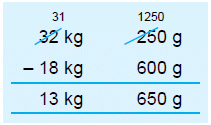Wheat consumed = 32 kg 250 g

Rice consumed = 18 kg 600 g

Difference in consumption of wheat and rice = 32 kg 250 g – 18 kg 600 g

Hence, required difference is 13 kg 650 g

Question 7: Mohan covered a distance of 15 km 275 m in the first hour and 18 km 803 m in the second hour. What distance did he cover in two hours?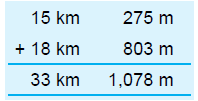Distance covered in first hour = 15 km 275 m.

Distance covered in second hour = 18 km 803 m

Total distance covered in 2 hours

= 15 km 275 m + 18 km 803 m

= 33 km + 1,000 m + 78 m = 34 km 78 m.

Hence, Mohan covered 34 km 78 m in two hours.

Question 8: Subtract :

(a) 7 km 207 m from 16 km 112 m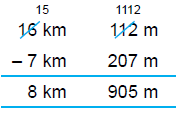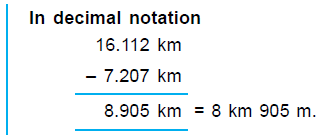(b) 10 m 72 cm from 42 m 63 cm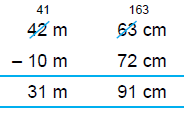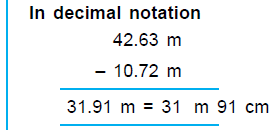(c) 26 kg 432 g from 51 kg 307 g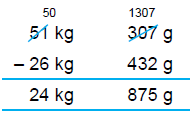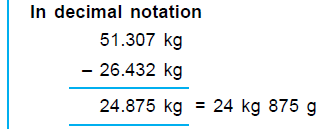(d) 11 l 853 ml from 44 l 92 ml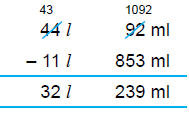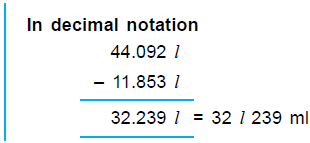(a) 10 km 525 m and 15 km 895 m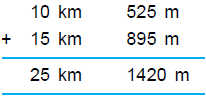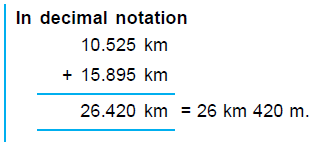= 25 km + 1,000 m + 420 m

= 25 km + 1 km + 420 m

= 26 km 420 m.

(b) 52 g 925 mg and 36 g 125 mg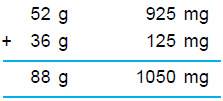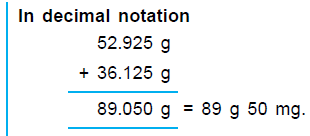= 88 g + 1,000 mg + 50 mg

= 88 g + 1 g + 50 mg = 89 g 50 mg.

(c) 16 kl 256 l and 27 kl 903 l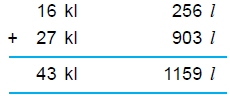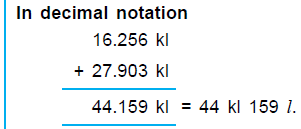= 43 kl + 1,000 l + 159 l

= 43 kl + 1 kl + 159 l = 44 kl 159 l.

(d) 71 cm 9 mm, 26 cm 3 mm and 9 mm.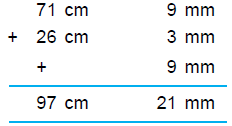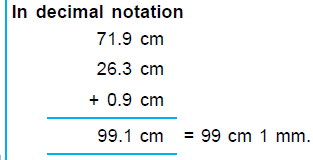= 97 cm + 20 mm + 1 mm

= 97 cm + 2 cm + 1 mm = 99 cm 1 mm.

Question 10: 7 410 ml soup was made. It was equally distributed among 6 persons. How much soup was given to each person?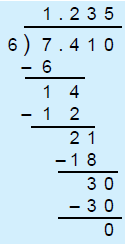Total quantity of soup = 7 410 ml = 7.410

Number of persons = 6

Soup given to each person = 7.410 ÷ 6

Hence, each person gets 1.235 = 1 235 ml soup.

Question 11: The length of ribbon required for making a design is 4 m 16 cm. What is the length of the ribbon required for making 8 such designs?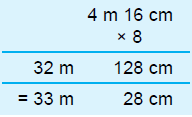Length of ribbon required for 1 design = 4 m 16 cm

Number of designs = 8

Total length of ribbon required = 4 m 16 cm × 8

Hence, required length of ribbon = 33 m 28 cm.

Question 12: Divide :

(a) 6 kg 128 g by 4

We can do the divisions by decimal notation as it is easier.

6 kg 128 g = 6.128 kg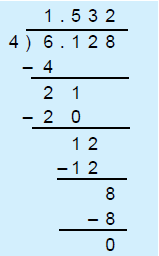Thus, Q uotient = 1.532 kg = 1 kg 532 g

Hence, 6 kg 128 g ÷ 4 = 1 kg 532 g.

(b) 7 m 53 cm by 3

7 m 53 cm = 7.53 m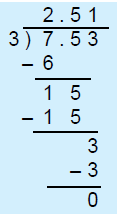Thus, quotient = 2.51 m = 2 m 51 cm

Hence, 7 m 53 cm ÷ 3 = 2 m 51 cm.

(c) 7 l 52 ml by 4

7 l 52 ml = 7.052 l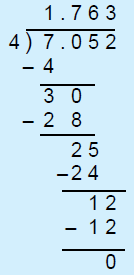Thus, quotient = 1.763 = 1 763 ml

Hence, 7 52 ml = 1 763 ml.

Question 13: Multiply :

(a) 4 km 365 m by 6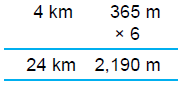= 24 km + 2,000 m + 190 m

= 24 km + 2 km + 190 m

= 26 km 190 m.

in decimal notation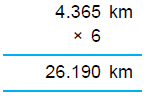= 26 km 190 m.

(b) 5 214 ml by 8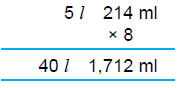= 40 + 1,000 ml + 712 ml

= 40 + 1 + 712 ml = 41 712 ml

in decimal notation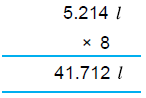= 41 712 ml.

(c) 18 kg 615 g by 5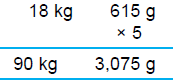= 90 kg + 3,000 g + 75 g

= 90 kg + 3 kg + 75 g = 93 kg 75 g.

in decimal notation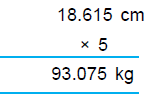= 93 kg 75 g.

(d) 18 cm 6 mm by 9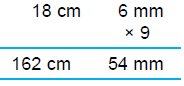= 162 cm + 50 mm + 4 mm

= 162 cm + 5 cm + 4 mm

= 167 cm 4 mm.

in decimal notation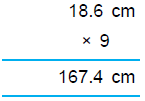The document Practice Questions with Solutions: Measurement of Length, Mass and Capacity - Notes | Study Mathematics for Class 5 - Class 5 is a part of the Class 5 Course Mathematics for Class 5.
All you need of Class 5 at this link: Class 5

## Mathematics for Class 5

12 videos|55 docs|35 tests
 Use Code STAYHOME200 and get INR 200 additional OFF

## Mathematics for Class 5

12 videos|55 docs|35 tests

Track your progress, build streaks, highlight & save important lessons and more!

,

,

,

,

,

,

,

,

,

,

,

,

,

,

,

,

,

,

,

,

,

,

,

,

;